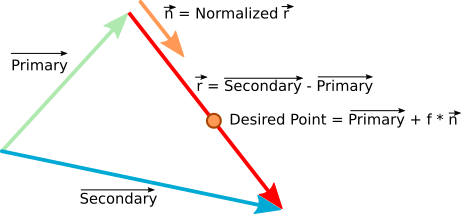# Finding a point between 2 Vector3() positionsAttention Topic was automatically imported from the old Question2Answer platform.Asked By Macryc

Hi All. I have 2 nodes (in 3D) and I need to find a position between them which is not just half-way from one to the other but closer to one or the other depending on other conditions.

Finding a midpoint between 2 vectors is kinda easy: sum them up and divide by 2.

But… What I need to do is to find a Vector3 that sits somewhere on the line connecting 2 other Vector3’s but its distance (on that line) from the primary vector3 to the secondary vector3 (ie, the 2 ends of the line) depends on the primary Vector3’s distance from a different node in the scene. I’ll call it the tertiary vector.

The data I have available is:

• Primary Vector3
• Secondary Vector3
• Distances between Primary, Secondary, and Tertiary Vector3’s

Eg, say the distance between primary and secondary Vector3 is 10. The distance between primary and tertiary is 100. In this case I want the looked-for Vector to be closer to the secondary vector (10ish units away from primary). But if the distance between primary and tertiary Vector3 is 1, I want the looked-for Vector to be 0.1 units away from the primary.Reply From: Ertain

Have you looked into interpolation, especially vector interpolation? Calculate how far the vectors need to be (use a ratio with a resulting quotient between 0.0 and 1.0) and use the `linear_interpolate()` function to find the desired `Vector3` object.Reply From: archeronSubtract the secondary from the primary vector. Call the resulting vector r. Now multiply r by any factor you want (0.5 would yield half the distance between the primary and the secondary), and add the resulting scaled vector r to the primary to get the desired position.

I’m not sure how your third vector influences the position of the desired point on r, since you gave only two examples, which aren’t enough to determine the function you have in mind, but it’s clear that the factor you scale r with depends on a simple calculation involving a constant value and the distance between the primary and the tertiary vector, which again you can get by a simple vector subtraction. Depending on whether the distance “d” between primary and tertiary can be potentially infinite or is at it’s maximum at 100, you need to use different function to map d to a value between 0 to 1 (the scaling factor).

If you want the factor f to move between 0 and 1, you scale the vector r; if you want the factor f to be something between 0 and the total distance between the primary and secondary heads, you can scale the normalized vector r instead - whatever is easier. Interpolation usually works with values between 0 and 1, though.Reply From: estebanmolca

Here I leave an example but it is in 2d, but so you can see the idea of what they answered previously:

``````extends Node2D

var a=Vector2(300,270)
var b=Vector2(640,350)
var c=Vector2(400,280)

func _draw():
draw_circle(a, 10, Color.red)
draw_circle(b, 10, Color.blue)
draw_circle(c, 10, Color.green)

var limits = a.distance_to(c) / a.distance_to(b)
var d = a.linear_interpolate(b, clamp(limits,0,1))
draw_circle(d, 10, Color.black)

func _process(delta):
c=get_local_mouse_position()
update()
pass
``````Reply From: WolframR

This is a bit of additional information, but you are looking for the mathmatical formula called the midpoint formula. You learn this in middle school, but inevitably forget it because who on earth is going to need to use it that often without a reason. There are plenty of formulas that come in handy in 2d and 3d coding, and even some in days handling, so it’ll help to keep that in mind and think about if a problem you are experiencing might be solved with something you learn in math class. Sometimes something might just pop out from memory and give you a lead to Google the details.